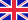•# Computational complexity theory

Autor: Source: Wikipedia

Source: Wikipedia. Pages: 94. Chapters: Quantum computer, Analysis of algorithms, Best, worst and average case, Communication complexity, Polynomial-time reduction, Interactive proof system, Complexity class, Time complexity, Aanderaa-Karp-Rosenberg conjecture,... Viac o knihe

Na objednávku, dodanie 2-4 týždne

21.00 €

bežná cena: 24.70 €

## O knihe

Source: Wikipedia. Pages: 94. Chapters: Quantum computer, Analysis of algorithms, Best, worst and average case, Communication complexity, Polynomial-time reduction, Interactive proof system, Complexity class, Time complexity, Aanderaa-Karp-Rosenberg conjecture, List-decoding, Universal hashing, Generic-case complexity, Randomness extractor, Information-based complexity, Pseudorandom generator theorem, Cook-Levin theorem, Klee-Minty cube, Computing the permanent, Unique games conjecture, Computational complexity of mathematical operations, Circuits over sets of natural numbers, Decision tree model, Approximation algorithm, Cobham's thesis, Turing reduction, Kernelization, Complexity index, Parameterized complexity, Circuit complexity, Context of computational complexity, Proof complexity, Karp-Lipton theorem, Computational topology, Propositional proof system, Combinatorial optimization, Nondeterministic algorithm, PCP theorem, Natural proof, Many-one reduction, Descriptive complexity theory, L-notation, Low, Savitch's theorem, Yao's principle, SO, Averaging argument, The Complexity of Songs, Strongly NP-complete, Interval-valued computation, Integer circuit, Configuration graph, Sparse language, Constructible function, Pseudo-polynomial time, Symmetric Turing machine, Pebble game, Schaefer's dichotomy theorem, Complement, L-reduction, Existential theory of the reals, Computational resource, Unary language, Electronic Colloquium on Computational Complexity, Boolean circuit, Log-space reduction, Advice, Smoothed analysis, Gap theorem, Switching lemma, Truth-table reduction, PTAS reduction, Dynamic problem, Asymptotic computational complexity, Semi-membership, Linear speedup theorem, Weakly NP-complete, Hardness of approximation, Blum's speedup theorem, Leaf language, Padding argument, Quantum complexity theory, Generalized game, Computation tree, Transdichotomous model, Compression theorem, Certificate, Effective complexity, Logical depth, Proper complexity function, Gadget, Introduction to the Theory of Computation. Excerpt: Computational complexity theory is a branch of the theory of computation in theoretical computer science and mathematics that focuses on classifying computational problems according to their inherent difficulty. In this context, a computational problem is understood to be a task that is in principle amenable to being solved by a computer (which basically means that the problem can be stated by a set of mathematical instructions). Informally, a computational problem consists of problem instances and solutions to these problem instances. For example, primality testing is the problem of determining whether a given number is prime or not. The instances of this problem are natural numbers, and the solution to an instance is yes or no based on whether the number is prime or not. A problem is regarded as inherently difficult if its solution requires significant resources, whatever the algorithm used. The theory formalizes this intuition, by introducing mathematical models of computation to study these problems and quantifying the amount of resources needed to solve them, such as time and storage. Other complexity measures are also used, such as the amount of communication (used in communication complexity), the number of gates in a circuit (used in circuit complexity) and the number of processors (used in parallel computing). One of the roles of computational complexity theory is to determine the practical limits on what co...

• Vydavateľstvo: Books LLC, Reference Series
• Rok vydania: 2011
• Formát: Paperback
• Rozmer: 246 x 189 mm
• Jazyk: Anglický jazyk
• ISBN: 9781156428269

Generuje redakčný systém BUXUS CMS spoločnosti ui42.